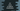# C# program to initialize an array of objects and iterate over the objects## C# program to initialize an array of objects:

In this post, we will learn how to initialize an array of objects in C#. We will create a class and different objects of that class. All of these objects will be inserted to an array.

With this program, you will learn how to create classes and how to initialize different objects of that class. We will also learn how to insert and iterate through these objects and print the values. If you already have an idea of classes and objects in C#, you will find it easier to understand.

Let’s write down the program.

### C# program to create an array of objects:

You can think a class like a blueprint that specifies what it can do. Objects are blocks of memories allocated based on the class blueprint. We can create multiple objects for a same class. These are also called instance of the class.

In this example, we will create one Student class with different properties to hold student info and we will create different objects of this class. Once all objects are created, the program will initialize an array with these objects.

We will also learn how to iterate through this array of objects.

### 2 different C# programs to create an array of objects:

Below C# program creates an array of objects:

``````namespace Program
{
public class Student
{
public string Name { get; set; }
public int Age { get; set; }
public char Grade { get; set; }
public Student(string name, int age, char grade)
{
Name = name;
Age = age;
}

public void PrintStudentInfo()
{
}
}

class Program
{
static void Main(string[] args)
{
Student[] StudentArray = { new Student("Alex", 21, 'A'), new Student("Bob", 22, 'B') };

StudentArray.PrintStudentInfo();
StudentArray.PrintStudentInfo();
}
}
}``````

Here,

• Student is a class that can hold three properties: Name, Age and Grade. Name is a string, Age is an integer and Grade is a character variable.
• In the constructor of the class, we are initializing it with the values of name, age and grade.
• The PrintStudentInfo method is printing the values of the Student class.
• One array, StudentArray is created with two Student objects. Both of these objects are initialized with different values.
• We are using the index values to access each of these items and calling PrintStudentInfo method to print the values.

If you run this program, it will print:

``````Name: Alex, Age: 21, Grade: A
Name: Bob, Age: 22, Grade: B``````

You can also create a new array of two items and assign values to each of these items separately.

``````namespace Program
{
public class Student
{
public string Name { get; set; }
public int Age { get; set; }
public char Grade { get; set; }
public Student(string name, int age, char grade)
{
Name = name;
Age = age;
}

public void PrintStudentInfo()
{
}
}

class Program
{
static void Main(string[] args)
{
Student[] StudentArray = new Student;

StudentArray = new Student("Alex", 21, 'A');
StudentArray = new Student("Bob", 22, 'B');

StudentArray.PrintStudentInfo();
StudentArray.PrintStudentInfo();
}
}
}``````

It will print a similar result.

### How to iterate through an array of objects:

We can use any loop to iterate through an array of objects and access the array element values. For example, the below program uses a foreach loop to iterate and print the details of each object:

``````namespace Program
{
public class Student
{
public string Name { get; set; }
public int Age { get; set; }
public char Grade { get; set; }
public Student(string name, int age, char grade)
{
Name = name;
Age = age;
}

public void PrintStudentInfo()
{
}
}

class Program
{
static void Main(string[] args)
{
Student[] StudentArray = { new Student("Alex", 21, 'A'), new Student("Bob", 22, 'B'), new Student("Daisy", 20, 'A') };

foreach (Student s in StudentArray)
{
s.PrintStudentInfo();
}
}
}
}``````

The StudentArray array holds three Student objects and the foreach loop iterate over the array and calls PrintStudentInfo method on each of these objects to print the details of each object.